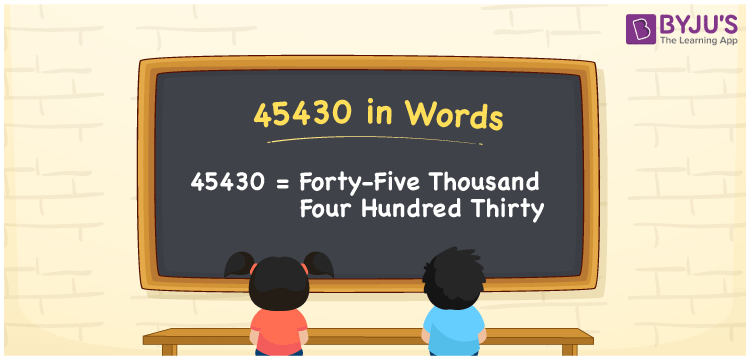# 45430 in words

45430 in words is written as Forty Five Thousand Four Hundred and Thirty. In 45430, 4 has a place value of ten thousand, 5 is in the place value of thousand, 4 is in the place value of hundred and 5 is in the place value of ten. The article on Place Value gives more information. The number 45430 is used in expressions that relate to money, distance, social media views, and many more. For example, “An extra comma in the code resulted in Forty Five Thousand Four Hundred and Thirty errors in a software program.”

 45430 in words Forty Five Thousand Four Hundred and Thirty Forty Five Thousand Four Hundred and Thirty in Numbers 45430

## 45430 in English Words## How to Write 45430 in Words?

We can convert 45430 to words using a place value chart. The number 45430 has 5 digits, so let’s make a chart that shows the place value up to 5 digits.

 Ten thousand Thousands Hundreds Tens Ones 4 5 4 3 0

Thus, we can write the expanded form as:

4 × Ten thousand + 5 × Thousand + 4 × Hundred + 3 × Ten + 0 × One

= 4 × 10000 + 5 × 1000 + 4 × 100 + 3 × 10 + 0 × 1

= 45430.

= Forty Five Thousand Four Hundred and Thirty.

45430 is the natural number that is succeeded by 45429 and preceded by 45431.

45430 in words – Forty Five Thousand Four Hundred and Thirty.

Is 45430 an odd number? – No.

Is 45430 an even number? – Yes.

Is 45430 a perfect square number? – No.

Is 45430 a perfect cube number? – No.

Is 45430 a prime number? – No.

Is 45430 a composite number? – Yes.

## Solved Example

1. Write the number 45430 in expanded form

Solution: 4 x 10000 + 5 x 1000 + 4 x 100 + 3 x 10 + 0 x 1

Or Just 4 x 10000 + 5 x 1000 + 4 x 100 + 3 x 10

We can write 45430 = 40000 + 5000 + 400 + 30 + 0

= 4 x 10000 + 5 x 1000 + 4 x 100 + 3 x 10 + 0 x 1.

## Frequently Asked Questions on 45430 in words

Q1

### How to write the number 45430 in words?

45430 in words is written as Forty Five Thousand Four Hundred and Thirty.
Q2

### State whether True or False. 45430 is divisible by 3?

False. 45430 is not divisible by 3.
Q3

### Is 45430 divisible by 10?

Yes. 45430 is divisible by 10.